## Tuesday, May 29, 2007

### Reduced Fractions

Hi, it's Ruby and Brenna; we will be talking about reduced fractions. To reduce a fraction you will need to divide by the greatest common factor. For example:

Let's say you have a fraction that is 10/25. You will need to find a number that will go into both numbers, 10 and 25. So what will go into both numbers? You guessed it, five. Five can go into ten two times and five can go into twenty-five, five times. So the answer would be 2/5 because 5 can go into 10, two times and five could go into twenty-five, five times.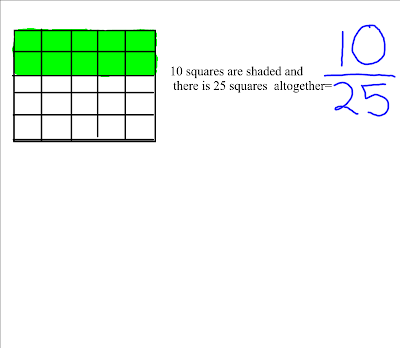Another example is:
Pretend you have a fraction that says 8/24 now reduce it. Find a number that will go into both numbers. Which number will go into both numbers? You guessed it again, eight. Eight can go into 8 once and 8 can go into 24 , 3 times. That would be 1/3.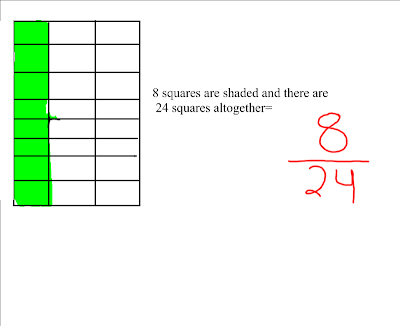## Friday, May 25, 2007

### Equivalent Fractions

Hey! Today we'll describe how to find equivalent fractions. Before we start we'll introduce. I am Il Han and my partner is Tai Hyun. Let's start.Il Han: You guys know fractions. Right.Tai Hyun: I like to play ...."Il Han: Stop introducing."Tai Hyun: Let's just say we have one out of quater and then if denominator changes to sixteen then numerator changes to what??You bet, if you don't get it then you might wanna listen very carefully. You guys know that 4 goes into 16 4 times which means you have to mutiply by four to the numerator. Every time if the numerator or denominator mutiplys or divide you have to do samething to numerator or denominator.

Another example:

Then I give you one more question. There was 5 out of 10. When 10 changes to 2 then 5 changes to what????You bet. You know why?? Because denominator(10) divided by 15 and that means you have to do samething to the numenator(5). So the answer is 1 out of 2 or half so this is it. Thanks for listening folks. Bye-bye.

## Tuesday, April 03, 2007

### Area of a Triangle

Hey, Jenna and Dillani here, we will be telling you how to find the area of a triangle. First, measure the base. Then, measure the height. Multiply base times height. But you’re not done!
You have to remember to divide by 2 because the triangle is ½ a rectangle or parallelogram. Here is an example of how to measure a triangle.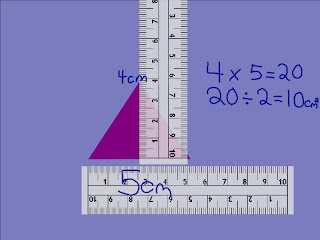## Wednesday, March 21, 2007

### Finding the Area of A Parallelogram

Hi, I'm Jessie, and this is my partner, Firoze. Firoze: That's FUR-OS-A. Sorry, she's very cautious when it comes to her name pronounciation. Okay, so, today we'll be showing you how to find the area of a parallelogram. Okay, so the first step is to measure the base or the width of the parallelogram.Next, measure the height or the length of the parallelogram. Remember, the side isn't the height. Imagine a dotted line from the top corners to the bottom, the line is straight, measure this imaginary line, not the side.Next you multiply the base by the height. As you can see, we've already started the equation.
Your answer should be 28 cm2. Noticted the 2 after the cm. This 2 stands for square. So your answer should be 28cm (squared).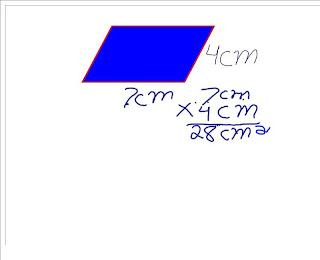Another way of finding the area of a parallelogram, is to put it on gragh paper. Count up al, the full squares that are in the parallelogram.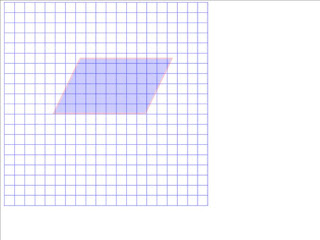Notice some squares aren't full. Try putting the half squares together, and counting them as one.
So that's basically it. It's very simple, we hope we made it clear to you. That's all for now. Firoze and Jessie signing off. We'll see you next time on 6BD' s math blog. Chouw for now!.